How to build polyhedra with paper and rubber bands

Cheap and simple technique to build a lot of polyhedra.Snub cube

In Dürer's book 'Underweysung der Messung' the author published the first plane net of polyhedra, for example, this snub cube:DodecahedronThe first drawing of a plane net of a regular dodecahedron was published by Dürer in his book 'Underweysung der Messung' ('Four Books of Measurement'), published in 1525 .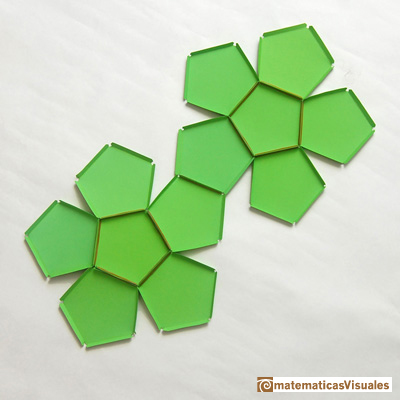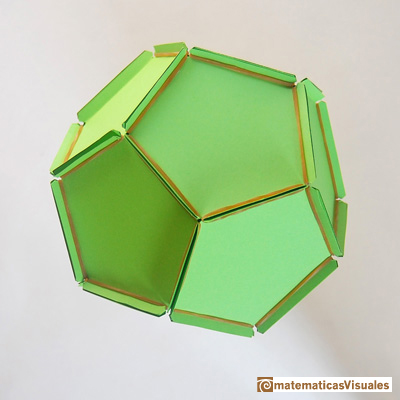CuboctahedronA cuboctahedron is an Archimedean solid. It can be seen as made by cutting off the corners of an octahedron.IcosidodecahedronTruncated TetrahedronThe truncated tetrahedron is an Archimedean solid made by 4 triangles and 4 hexagons.Durer was the first who publish a plane net of a truncated tetrahedron:Truncated CubeWhen you truncate a cube you get a truncated cube and a cuboctahedron. If you truncate an octahedron you get a truncated octahedron and a cuboctahedron.

You need six octogons and eight equilateral triangles: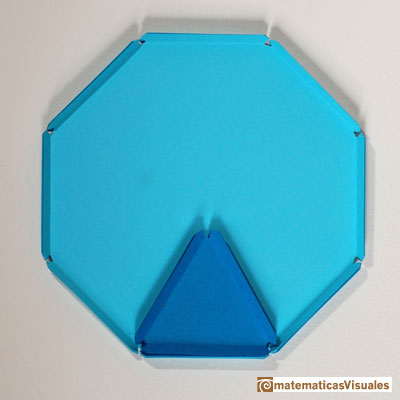This is the plane net of a truncated cube: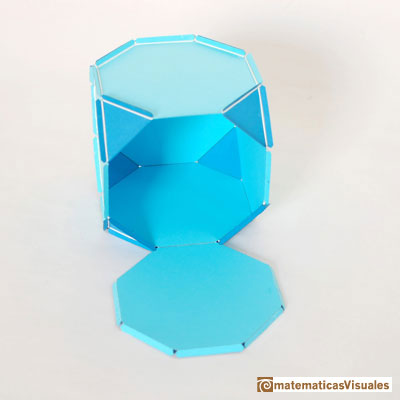Icosahedral lamp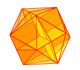The twelve vertices of an icosahedron lie in three golden rectangles. Then we can calculate the volume of an icosahedron

Very easy icosahedral lamp built with cardboard and rubber bands: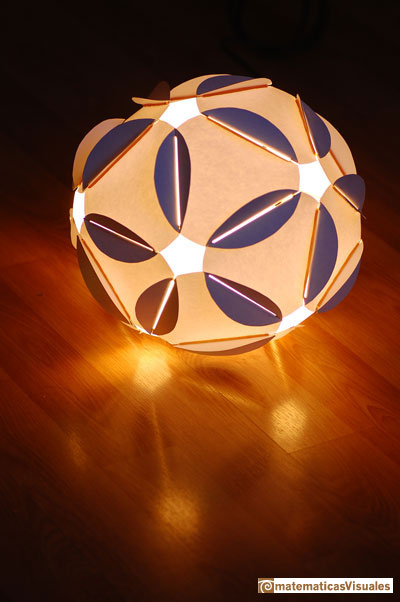More: dannish lamp (IQlight):This model was designed by Holger Strom and it is called IQlight. It is based in the rhombic triacontahedon.

REFERENCES

Magnus Wenninger - 'Polyhedron Models', Cambridge University Press.
Hugo Steinhaus - Mathematical Snapshots - Oxford University Press - Third Edition (p. 197)
Peter R. Cromwell - 'Polyhedra', Cambridge University Press, 1999.
H.Martin Cundy and A.P. Rollet, 'Mathematical Models', Oxford University Press, Second Edition, 1961 (p. 87).
W.W. Rouse Ball and H.S.M. Coxeter - 'Matematical Recreations & Essays', The MacMillan Company, 1947.Simple technique to build polyhedra gluing discs made of cardboard or paper.Italian designer Bruno Munari conceived 'Acona Biconbi' as a work of sculpture. It is also a beautiful game to play with colors and shapes.Material for a session about polyhedra (Zaragoza, 9th May 2014). Simple techniques to build polyhedra like the tetrahedron, octahedron, the cuboctahedron and the rhombic dodecahedron. We can build a box that is a rhombic dodecahedron.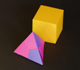Material for a session about polyhedra (Zaragoza, 7th November 2014). We study the octahedron and the tetrahedron and their volumes. The truncated octahedron helps us to this task. We build a cubic box with cardboard and an origami tetrahedron.Material for a session about polyhedra (Zaragoza, 23rd Octuber 2015) . Building a cube with cardboard and an origami octahedron.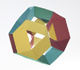Material for a session about polyhedra (Zaragoza, 21st October 2016). Instructions to build several geometric bodies.Some properties of this platonic solid and how it is related to the golden ratio. Constructing dodecahedra using different techniques.The first drawing of a plane net of a regular dodecahedron was published by Dürer in his book 'Underweysung der Messung' ('Four Books of Measurement'), published in 1525 .A cuboctahedron is an Archimedean solid. It can be seen as made by cutting off the corners of a cube.The truncated tetrahedron is an Archimedean solid made by 4 triangles and 4 hexagons.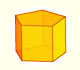We study different prisms and we can see how they develop into a plane net. Then we explain how to calculate the lateral surface area.We study different cylinders and we can see how they develop into a plane. Then we explain how to calculate the lateral surface area.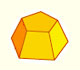Plane net of pyramids and pyramidal frustrum. How to calculate the lateral surface area.Plane developments of cones and conical frustum. How to calculate the lateral surface area.The first drawing of a plane net of a regular dodecahedron was published by Dürer in his book 'Underweysung der Messung' ('Four Books of Measurement'), published in 1525 .